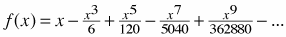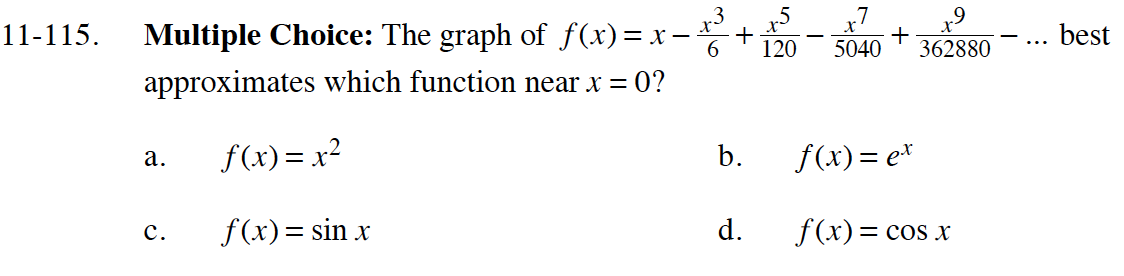### Home > CALC > Chapter 11 > Lesson 11.3.2 > Problem11-115

11-115.
1. Multiple Choice: The graph ofbest approximates which function near x = 0? Homework Help ✎

1. f(x) = x2

2. f(x) = ex

3. f(x) = sin x

4. f(x) = cos xNotice that the given polynomial function is odd.